Function Repository Resource:

# CanalSurface

Compute the parametrization of a canal surface

Contributed by: Wolfram Staff (original content by Alfred Gray)
 ResourceFunction["CanalSurface"][dir,pm,r,{t,θ}] computes the parametrization of a canal surface of variable radius r and parameter pm with directrix dir and variables t,θ.

## Details and Options

A channel or canal surface is a surface generated as the envelope of a one-parameter family of spheres with respect to a directrix.

## Examples

### Basic Examples (2)

Define a circle in 3D:

 In:=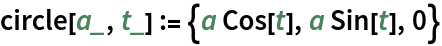Compute a canal surface, using the circle as the directrix:

 In:=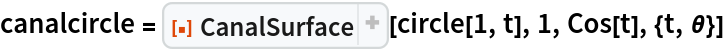Out=Plot the resulting canal surface:

 In:=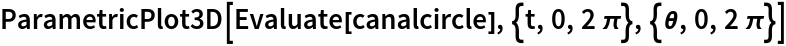Out=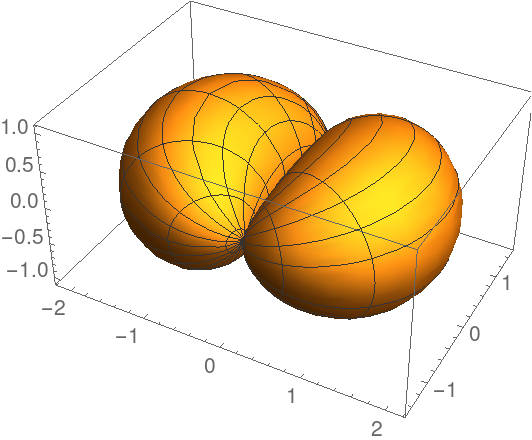Plot the circle and a canal surface, along with the spheres:

 In:=Out=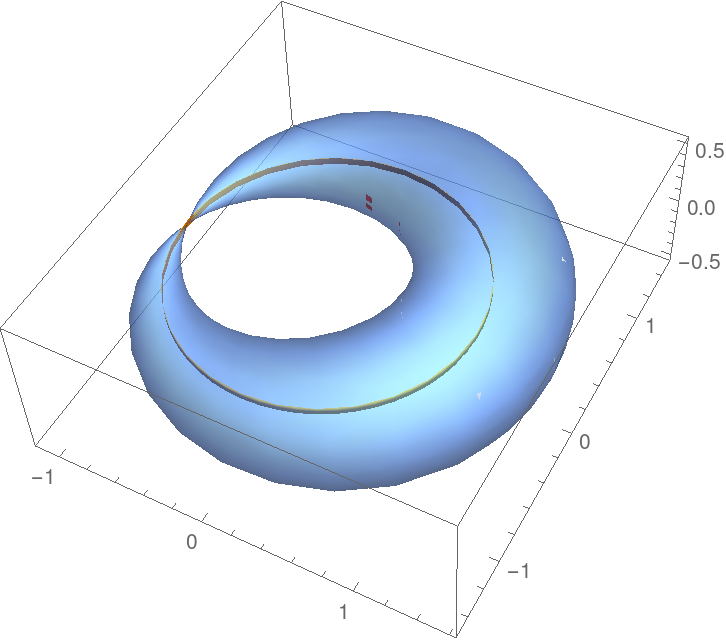Define a helix in 3D:

 In:=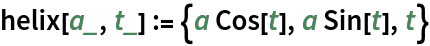Compute a canal surface, using the helix as the directrix:

 In:=Out=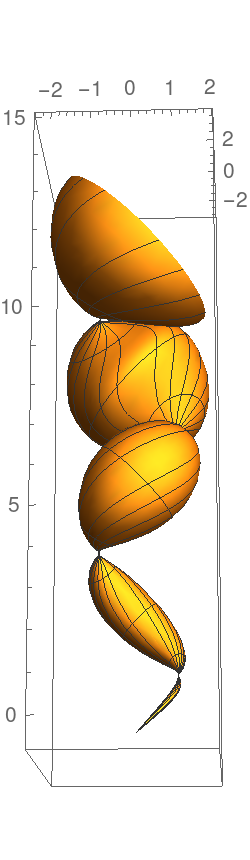Enrique Zeleny

## Version History

• 1.0.0 – 26 February 2020